Next: Expansion of Tide Generating Up: Terrestrial Ocean Tides Previous: Tide Generating Potential

# Decomposition of Tide Generating Potential

Let,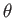,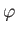be right-handed spherical coordinates in a non-rotating reference frame whose origin lies at the center of the planet, and whose symmetry axis coincides with the planetary rotation axis. Thus, the vector position of a general point is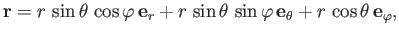(12.15)

where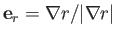, et cetera. Let the coordinates of the moon's center be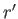,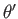,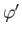. It follows that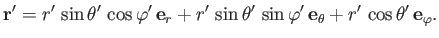(12.16)

Hence, from Equation (12.5),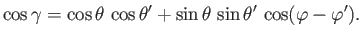(12.17)

Now, according to the spherical harmonic addition theorem (Arfken 1985),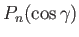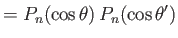(12.18)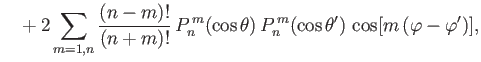(12.19)

which implies that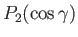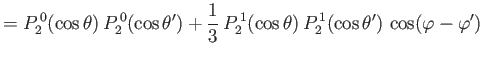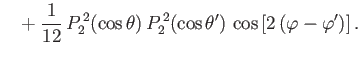(12.20)

Here,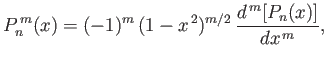(12.21)

for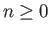and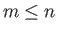, denotes an associated Legendre function (Abramowitz and Stegun 1965). In particular,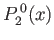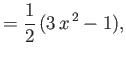(12.22)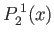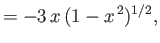(12.23)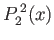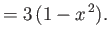(12.24)

Note that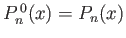.

Let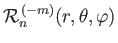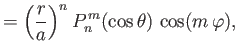(12.25)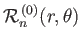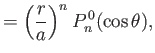(12.26)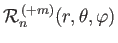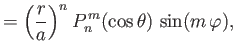(12.27)

where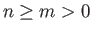. According to Equations (12.14), (12.20), and (12.25)-(12.27),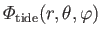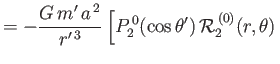(12.28)

Here, we have neglected the unimportant constant term in Equation (12.14).Next: Expansion of Tide Generating Up: Terrestrial Ocean Tides Previous: Tide Generating Potential
Richard Fitzpatrick 2016-03-31